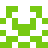### Real Vim ninjas count every keystroke - do you?

###### Pick a challenge, fire up Vim, and show us what you got.

```Your VimGolf key: please sign in

\$ gem install vimgolf
\$ vimgolf setup
\$ vimgolf put 9v0060da5177000000000209
```

### Plotting some variables in python

4 sets of (x,y) variables to plot. Just increment the numbers! Well, let's change the colors of the lines as well. Oh and we meant to plot the absolute values of course.

##### Start file
```def Plot_All():
plt.plot(x1,y1,color='k',lw=0.8,label="line #1")
plt.plot(x1,y1,color='k',lw=0.8,label="line #1")
plt.plot(x1,y1,color='k',lw=0.8,label="line #1")
plt.plot(x1,y1,color='k',lw=0.8,label="line #1")
```
##### End file
```def Plot_All():
plt.plot(x1,abs(y1),color='k',lw=0.8,label="line #1")
plt.plot(x2,abs(y2),color='b',lw=0.8,label="line #2")
plt.plot(x3,abs(y3),color='r',lw=0.8,label="line #3")
plt.plot(x4,abs(y4),color='g',lw=0.8,label="line #4")
```

#### View Diff

```2,5c2,5
<     plt.plot(x1,y1,color='k',lw=0.8,label="line #1")
<     plt.plot(x1,y1,color='k',lw=0.8,label="line #1")
<     plt.plot(x1,y1,color='k',lw=0.8,label="line #1")
<     plt.plot(x1,y1,color='k',lw=0.8,label="line #1")
---
>     plt.plot(x1,abs(y1),color='k',lw=0.8,label="line #1")
>     plt.plot(x2,abs(y2),color='b',lw=0.8,label="line #2")
>     plt.plot(x3,abs(y3),color='r',lw=0.8,label="line #3")
>     plt.plot(x4,abs(y4),color='g',lw=0.8,label="line #4")
```

### Solutions by @AAbraxas1:

Unlock 7 remaining solutions by signing in and submitting your own entry

## 305 active golfers, 1431 entries

##### Solutions by @AAbraxas1:34
###### #3 - AAbraxas / @AAbraxas1

07/01/2021 at 08:12PM35
###### #>50 - AAbraxas / @AAbraxas1

07/01/2021 at 08:08PM36
###### #>74 - AAbraxas / @AAbraxas1

07/01/2021 at 08:05PM39
###### #>95 - AAbraxas / @AAbraxas1

07/01/2021 at 07:58PM45
###### #>144 - AAbraxas / @AAbraxas1

07/01/2021 at 07:51PM49
###### #>169 - AAbraxas / @AAbraxas1

07/01/2021 at 07:47PM57
###### #>212 - AAbraxas / @AAbraxas1

07/01/2021 at 07:39PM